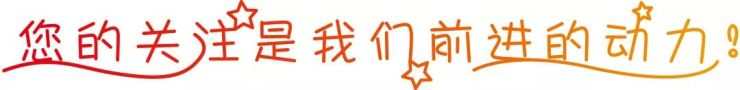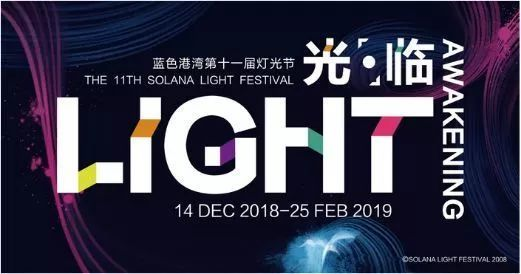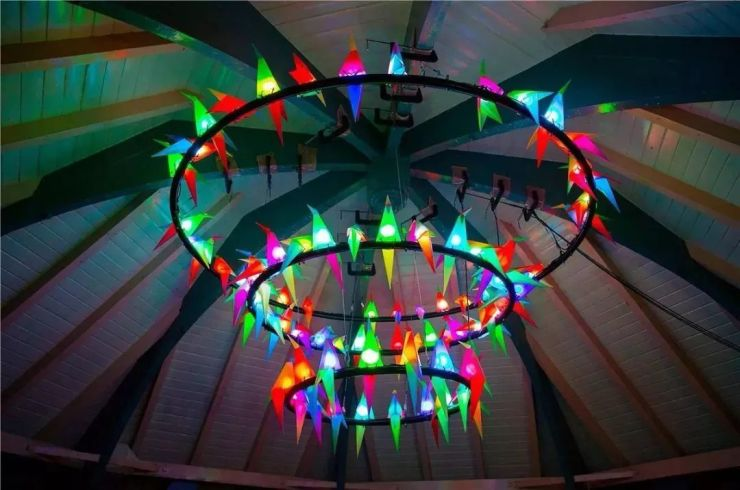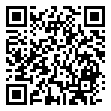|

# 蓝色港湾灯光节“光·临”2019年第11届蓝色港湾灯光节“光·临”，于2018年12月14日在朝阳公园蓝色港湾拉开帷幕，不负冬日的流光溢彩，一同体验前所未见的光影未来吧！连续74天1776小时不间断点亮北京夜空，屡获国际大奖的灯光艺术装置，融合东方哲学意蕴，跨界延伸城市公共艺术空间的无限可能……蓝色港湾灯光节已成功举办十年，每一届灯光节都会在细节和创意上精雕细琢，在多样化和趣味性上大胆尝试，在互动性和参与感上颠覆想象，极大地提升了大众对城市艺术空间的认知度和认同感。

经过数月虔诚探寻世界各角落的光影艺术作品后，蓝色港湾甄选来自德国和澳大利亚的灯光艺术装置将于12月14日揭开神秘的面纱——《STALACTITE》(钟乳石)、《OCTOPODA》(八爪鱼)、《PARROT PARTY》(鹦鹉派对)……

这些光影互动艺术作品首次由蓝色港湾引入中国，这不是简单地空运复制，而是将东西方艺术文化进行融合创新，绝对颠覆你的想象!

1、《STALACTITE》(钟乳石)是来自2018年比利时布鲁塞尔灯光节(BRIGHT BRUSSELSFESTIVAL)的巨大灯光艺术装置，整体结构重量达2.3吨。该作品是由德国红点奖IF 奖得主克里斯托弗(Christopher M.Bauder) 创作，他亦是与张艺谋导演共同完成《对话·寓言2047》整体舞台灯光作品的艺术家。

每位现场的观众站在装置下方，就如同钟乳石上掉下来的水珠，伴随着音乐一起，共同构成整个装置艺术作品的动态组成部分。2、灯光艺术装置《OCTOPODA》(八爪鱼 )是来自新加坡I Light MarinaBay滨海湾灯光节的作品，是由一家来自悉尼的以工业设计为背景的阿米戈艺术工作室(Amigo and Amigo)的作品。阿米戈工作室由艺术家西蒙娜(Simone Chua)主导，她每一个项目中都能创作出将观众聚集在公共空间并且吸引观众想象力的作品。

《OCTOPODA》(八爪鱼)是一种蒸汽朋克打击乐结构的八爪鱼造型艺术装置，非常适用于所有年龄层的人群互动。它以八支触须鼓为特色，每只触须敲鼓时，都会发出一种独特的颜色，并随着鼓声闪烁。当八个鼓一起演奏的时候，《OCTOPODA》(八爪鱼 )会用他的机械动作和有节奏的灯光表演让观众眼花缭乱。

3、受到极度濒危的新西兰鹦鹉启发，创作者扎拉(Zara Pasfield)希望《PARROTPARTY》(鹦鹉派对)能提高人们对这些独特鸟类脆弱处境的认识。这些鹦鹉的结构内部被灯光点亮，使用折叠几何图形创建出鸟的自然形状，身上呈现出活力四射的橙色、蓝色和绿色。它们栖息在穹顶上，当周围有人的时候，它们就高声歌唱，变得高兴又兴奋并发出五彩缤纷的光。《PARROT PARTY》(鹦鹉派对)的创作者扎拉(ZaraPasfield)是一位才华横溢充满能量的灯光艺术家。在2017年从UTS获得空间建筑学学位后，她在短时间内参加了世界各地的主要灯光节日，包括2018年带着作品《PARROTPARTY》(鹦鹉派对)参加了悉尼(vivid Sydney)灯光节。开幕之日，蓝色港湾特邀中国当代艺术家郝方圆，在现场将进行传统乐器的演奏，并融合绘画、音乐、行为、影像、动画以及配合电子音乐效果，呈现全新多元化艺术感官体验。

无论是人生的幸福圆满，抑或宇宙自然的原理真相，无不受到东方文明的启迪与影响。郝方圆现场进行东方哲学的绘画演绎，抒写现实与虚拟，现在与未来相融相织，带你了解这个多时空多维度的幻彩世界。艺术是心灵的捕手，好的艺术具有高度的精神价值，能促进人与人，人与自然、社会的交流与和谐。

十年前，SOLANA蓝色港湾便开启了城市空间艺术的探索和传承之旅，将一件件具有高度审美价值的艺术品接入商业空间中，通过灯光节将文化艺术融入大众生活中，在照亮整个蓝色港湾的同时，更赋予了北京这座城市超级想象力和幸福感。那么，介绍了这么多有人会问，小编呀！我们以前没有去过，该怎么去呢？下面小编就把本次灯光节的交通路线告诉大家

677路985路枣营北里站下，向北200米即到或419路682路621路枣营路北口下车即到。

402路413路418路420路503路659路688路701路955路909路运通107路安家楼站洗车，行至朝阳公园路(好运街)向南300米即到。

`声明：本文由入驻焦点开放平台的作者撰写，除焦点官方账号外，观点仅代表作者本人，不代表焦点立场错误信息举报电话： 400-099-0099，邮箱：jubao@vip.sohu.com，或点此进行意见反馈，或点此进行举报投诉。`A B C D E F G H J K L M N P Q R S T W X Y Z
A - B - C - D - E
• A
• 鞍山
• 安庆
• 安阳
• 安顺
• 安康
• 澳门
• B
• 北京
• 保定
• 包头
• 巴彦淖尔
• 本溪
• 蚌埠
• 亳州
• 滨州
• 北海
• 百色
• 巴中
• 毕节
• 保山
• 宝鸡
• 白银
• 巴州
• C
• 承德
• 沧州
• 长治
• 赤峰
• 朝阳
• 长春
• 常州
• 滁州
• 池州
• 长沙
• 常德
• 郴州
• 潮州
• 崇左
• 重庆
• 成都
• 楚雄
• 昌都
• 慈溪
• 常熟
• D
• 大同
• 大连
• 丹东
• 大庆
• 东营
• 德州
• 东莞
• 德阳
• 达州
• 大理
• 德宏
• 定西
• 儋州
• 东平
• E
• 鄂尔多斯
• 鄂州
• 恩施
F - G - H - I - J
• F
• 抚顺
• 阜新
• 阜阳
• 福州
• 抚州
• 佛山
• 防城港
• G
• 赣州
• 广州
• 桂林
• 贵港
• 广元
• 广安
• 贵阳
• 固原
• H
• 邯郸
• 衡水
• 呼和浩特
• 呼伦贝尔
• 葫芦岛
• 哈尔滨
• 黑河
• 淮安
• 杭州
• 湖州
• 合肥
• 淮南
• 淮北
• 黄山
• 菏泽
• 鹤壁
• 黄石
• 黄冈
• 衡阳
• 怀化
• 惠州
• 河源
• 贺州
• 河池
• 海口
• 红河
• 汉中
• 海东
• 怀来
• I
• J
• 晋中
• 锦州
• 吉林
• 鸡西
• 佳木斯
• 嘉兴
• 金华
• 景德镇
• 九江
• 吉安
• 济南
• 济宁
• 焦作
• 荆门
• 荆州
• 江门
• 揭阳
• 金昌
• 酒泉
• 嘉峪关
K - L - M - N - P
• K
• 开封
• 昆明
• 昆山
• L
• 廊坊
• 临汾
• 辽阳
• 连云港
• 丽水
• 六安
• 龙岩
• 莱芜
• 临沂
• 聊城
• 洛阳
• 漯河
• 娄底
• 柳州
• 来宾
• 泸州
• 乐山
• 六盘水
• 丽江
• 临沧
• 拉萨
• 林芝
• 兰州
• 陇南
• M
• 牡丹江
• 马鞍山
• 茂名
• 梅州
• 绵阳
• 眉山
• N
• 南京
• 南通
• 宁波
• 南平
• 宁德
• 南昌
• 南阳
• 南宁
• 内江
• 南充
• P
• 盘锦
• 莆田
• 平顶山
• 濮阳
• 攀枝花
• 普洱
• 平凉
Q - R - S - T - W
• Q
• 秦皇岛
• 齐齐哈尔
• 衢州
• 泉州
• 青岛
• 清远
• 钦州
• 黔南
• 曲靖
• 庆阳
• R
• 日照
• 日喀则
• S
• 石家庄
• 沈阳
• 双鸭山
• 绥化
• 上海
• 苏州
• 宿迁
• 绍兴
• 宿州
• 三明
• 上饶
• 三门峡
• 商丘
• 十堰
• 随州
• 邵阳
• 韶关
• 深圳
• 汕头
• 汕尾
• 三亚
• 三沙
• 遂宁
• 山南
• 商洛
• 石嘴山
• T
• 天津
• 唐山
• 太原
• 通辽
• 铁岭
• 泰州
• 台州
• 铜陵
• 泰安
• 铜仁
• 铜川
• 天水
• 天门
• W
• 乌海
• 乌兰察布
• 无锡
• 温州
• 芜湖
• 潍坊
• 威海
• 武汉
• 梧州
• 渭南
• 武威
• 吴忠
• 乌鲁木齐
X - Y - Z
• X
• 邢台
• 徐州
• 宣城
• 厦门
• 新乡
• 许昌
• 信阳
• 襄阳
• 孝感
• 咸宁
• 湘潭
• 湘西
• 西双版纳
• 西安
• 咸阳
• 西宁
• 仙桃
• 西昌
• Y
• 运城
• 营口
• 盐城
• 扬州
• 鹰潭
• 宜春
• 烟台
• 宜昌
• 岳阳
• 益阳
• 永州
• 阳江
• 云浮
• 玉林
• 宜宾
• 雅安
• 玉溪
• 延安
• 榆林
• 银川
• Z
• 张家口
• 镇江
• 舟山
• 漳州
• 淄博
• 枣庄
• 郑州
• 周口
• 驻马店
• 株洲
• 张家界
• 珠海
• 湛江
• 肇庆
• 中山
• 自贡
• 资阳
• 遵义
• 昭通
• 张掖
• 中卫

1室1厅1厨1卫1阳台

1
2
3
4
5

0
1
2

1

1

0
1
2
3报名成功，资料已提交审核A B C D E F G H J K L M N P Q R S T W X Y Z
A - B - C - D - E
• A
• 鞍山
• 安庆
• 安阳
• 安顺
• 安康
• 澳门
• B
• 北京
• 保定
• 包头
• 巴彦淖尔
• 本溪
• 蚌埠
• 亳州
• 滨州
• 北海
• 百色
• 巴中
• 毕节
• 保山
• 宝鸡
• 白银
• 巴州
• C
• 承德
• 沧州
• 长治
• 赤峰
• 朝阳
• 长春
• 常州
• 滁州
• 池州
• 长沙
• 常德
• 郴州
• 潮州
• 崇左
• 重庆
• 成都
• 楚雄
• 昌都
• 慈溪
• 常熟
• D
• 大同
• 大连
• 丹东
• 大庆
• 东营
• 德州
• 东莞
• 德阳
• 达州
• 大理
• 德宏
• 定西
• 儋州
• 东平
• E
• 鄂尔多斯
• 鄂州
• 恩施
F - G - H - I - J
• F
• 抚顺
• 阜新
• 阜阳
• 福州
• 抚州
• 佛山
• 防城港
• G
• 赣州
• 广州
• 桂林
• 贵港
• 广元
• 广安
• 贵阳
• 固原
• H
• 邯郸
• 衡水
• 呼和浩特
• 呼伦贝尔
• 葫芦岛
• 哈尔滨
• 黑河
• 淮安
• 杭州
• 湖州
• 合肥
• 淮南
• 淮北
• 黄山
• 菏泽
• 鹤壁
• 黄石
• 黄冈
• 衡阳
• 怀化
• 惠州
• 河源
• 贺州
• 河池
• 海口
• 红河
• 汉中
• 海东
• 怀来
• I
• J
• 晋中
• 锦州
• 吉林
• 鸡西
• 佳木斯
• 嘉兴
• 金华
• 景德镇
• 九江
• 吉安
• 济南
• 济宁
• 焦作
• 荆门
• 荆州
• 江门
• 揭阳
• 金昌
• 酒泉
• 嘉峪关
K - L - M - N - P
• K
• 开封
• 昆明
• 昆山
• L
• 廊坊
• 临汾
• 辽阳
• 连云港
• 丽水
• 六安
• 龙岩
• 莱芜
• 临沂
• 聊城
• 洛阳
• 漯河
• 娄底
• 柳州
• 来宾
• 泸州
• 乐山
• 六盘水
• 丽江
• 临沧
• 拉萨
• 林芝
• 兰州
• 陇南
• M
• 牡丹江
• 马鞍山
• 茂名
• 梅州
• 绵阳
• 眉山
• N
• 南京
• 南通
• 宁波
• 南平
• 宁德
• 南昌
• 南阳
• 南宁
• 内江
• 南充
• P
• 盘锦
• 莆田
• 平顶山
• 濮阳
• 攀枝花
• 普洱
• 平凉
Q - R - S - T - W
• Q
• 秦皇岛
• 齐齐哈尔
• 衢州
• 泉州
• 青岛
• 清远
• 钦州
• 黔南
• 曲靖
• 庆阳
• R
• 日照
• 日喀则
• S
• 石家庄
• 沈阳
• 双鸭山
• 绥化
• 上海
• 苏州
• 宿迁
• 绍兴
• 宿州
• 三明
• 上饶
• 三门峡
• 商丘
• 十堰
• 随州
• 邵阳
• 韶关
• 深圳
• 汕头
• 汕尾
• 三亚
• 三沙
• 遂宁
• 山南
• 商洛
• 石嘴山
• T
• 天津
• 唐山
• 太原
• 通辽
• 铁岭
• 泰州
• 台州
• 铜陵
• 泰安
• 铜仁
• 铜川
• 天水
• 天门
• W
• 乌海
• 乌兰察布
• 无锡
• 温州
• 芜湖
• 潍坊
• 威海
• 武汉
• 梧州
• 渭南
• 武威
• 吴忠
• 乌鲁木齐
X - Y - Z
• X
• 邢台
• 徐州
• 宣城
• 厦门
• 新乡
• 许昌
• 信阳
• 襄阳
• 孝感
• 咸宁
• 湘潭
• 湘西
• 西双版纳
• 西安
• 咸阳
• 西宁
• 仙桃
• 西昌
• Y
• 运城
• 营口
• 盐城
• 扬州
• 鹰潭
• 宜春
• 烟台
• 宜昌
• 岳阳
• 益阳
• 永州
• 阳江
• 云浮
• 玉林
• 宜宾
• 雅安
• 玉溪
• 延安
• 榆林
• 银川
• Z
• 张家口
• 镇江
• 舟山
• 漳州
• 淄博
• 枣庄
• 郑州
• 周口
• 驻马店
• 株洲
• 张家界
• 珠海
• 湛江
• 肇庆
• 中山
• 自贡
• 资阳
• 遵义
• 昭通
• 张掖
• 中卫• 手机• 分享
• 设计
免费设计
• 计算器
装修计算器
• 入驻
合作入驻
• 联系
联系我们
• 置顶
返回顶部